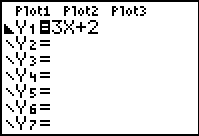# Knowledge Base

## Solution 11966: Graphing Inequalities on the TI-83 Plus and TI-84 Plus.

### How do I graph inequalities on the TI-83 Plus and TI-84 Plus?

The Y= Editor does not allow an inequality to be directly entered into the calculator. However, the graph styles feature can be utilized to show the solution set for an inequality. The example below will demonstrate how to graph a simple inequality using these features.

Example: Graph y<=3x+2

1) Press the [Y=] key For Y1=, input 3x+2.

2) Repeatedly press the left arrow key until the symbol to the left of Y1 is flashing. Press the [ENTER] key repeatedly until a triangle pointing to the lower part is displayed. This will change the graph style to shade below the function (this style was chosen because the solution set consists of all real numbers below the line of the y= equation).

3) Press the [GRAPH]  key to graph the solution set.The Inequality Graphing application can also be used to graph this solution.

Please see the TI-83 Plus and TI-84 Plus guidebooks for additional information.